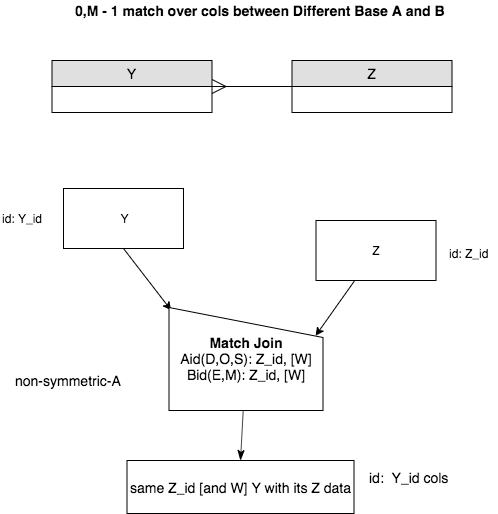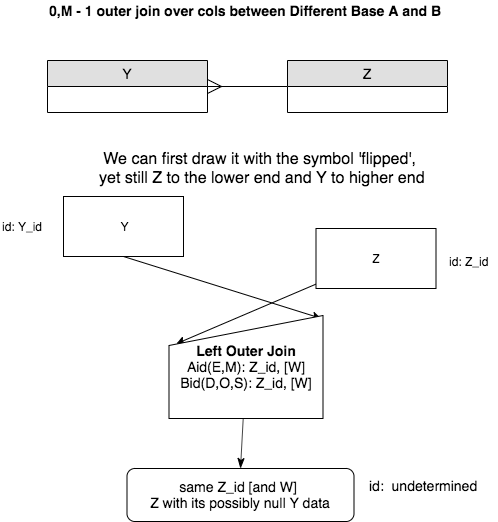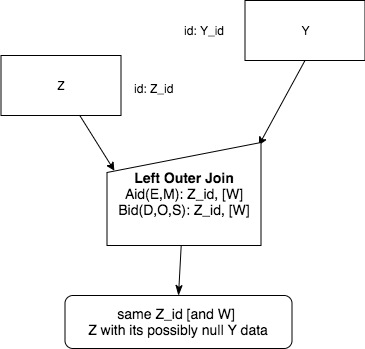# 14.2. A Generic Version of OuterJoin¶

Outer Join is used in the case where we have a 1 - M relationship and the many end relation (B) can have zero related foreign key data values from the relation on the one end (A). (Note A, B are swapped from the natural join case.)

Here are some generic versions of the precedence charts. First, the normal natural join.Now, when joining over at least the foreign key, but keeping all elements from the one-end A relation and adding NULL values from the many end B relation, we perform a Left Outer Join like this as an intermediate step:Then we finish by fully flipping the A (1 end) and B (0, M end) in the diagram like this:As we saw in the examples in the previous section, we can include added columns besides the identifier of the A relation, which we have called Z in these generic charts. This is shown with the [W] for additional column names.

Note

The Aid(…) and Bid(…) possible values have flipped from the natural match join to the left outer join. This is because the one-end relation is now the A relation and the zero or many end relation is now the B relation, but we match over the same columns that we did for the natural join.